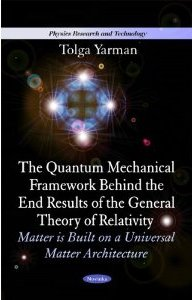Enter the content which will be displayed in sticky bar

# BookPages: 63
Publisher: Nova Science Publishers, Inc.
Year: 2010
ISBN: 1617613185
ISBN: 978-1617613180

Quantum Mechanical Framework Behind the End Results of the General Theory of Relativity (Physics Research and Technology) (Buy Now)

This book provides a new approach toward the end results of the General Theory of Relativity (GTR), based on the law conservation of energy, though in the broader sense of the concept of \'\'energy\'\', thus embodying the mass and energy equivalence of the Special Theory of Relativity (STR). Thereby, the approach is solely based on this latter theory (excluding the necessity of assuming the \'\'principle of equivalence\'\' of the GTR). The author provides a theorem which basically says that if in a relativistic or non-relativistic quantum mechanical description, composed properly, the mass of the object at hand is multiplied by an arbitrary number, then the total energy of it, is multiplied by, and its size is divided by this number. This book presents data which concludes that the quantum mechanical invariance obtained between total energy, mass and size - with respect to a mass change introduced in the original wave-like description of the object - is the fundamental basis of a universal matter architecture (UMA), and insures the end results of the GTR.

Preface

Chapter 1. INTRODUCTION, pp. 1-2

Chapter 2. BRIEF SKETCH OF THE IDEA, pp. 3-16

Chapter 3. RECAPITULATION: EXPECTED OCCURRENCES ABOUT A PARTICLE STATICALLY BOUND TO A FIELD, pp. 17-18

Chapter 4. DISCUSSION ABOUT THE CONCEPT OF FIELD: THE CLASSICAL COULOMB?S LAW, OR NEWTON?S LAW REIGNS IN BETWEEN, EXCLUSIVELY, STATIC CHARGES AND STATIC MASSES, RESPECTIVELY, WHILE THEIR 1/r**2 DEPENDENCY, IS A REQUIREMENT IMPOSED BY THE SPECIAL THEORY OF RELATIVITY, pp. 19-21

Chapter 5. GENERAL QUANTUM MECHANICAL THEOREM: THE QUANTITY (TOTAL ENERGY) x (CLOCK MASS) x (CLOCK SPACE SIZE)**2}, COMPOSED WITH RESPECT TO AN OBJECT, TURNS OUT TO BE A UNIVERSAL INVARIANT STRAPPED TO THE SQUARE OF THE PLANCK CONSTANT, pp. 23-35

Chapter 6. CONCLUSION, pp. 37-40

Appendix A.
Appendix B.
Appendix C.

Acknowledgments
References
Index